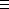#### You may also like### Rule of Three

If it takes four men one day to build a wall, how long does it take 60,000 men to build a similar wall?### Crossing the Atlantic

Every day at noon a boat leaves Le Havre for New York while another boat leaves New York for Le Havre. The ocean crossing takes seven days. How many boats will each boat cross during their journey?### On Time

On a clock the three hands - the second, minute and hour hands - are on the same axis. How often in a 24 hour day will the second hand be parallel to either of the two other hands?

# Friday 13th

##### Age 11 to 14Challenge Level

There were three Friday 13 ths in 1998, this is the greatest number that can occur in any one year, and there must be at least one each year.

Here is the solution from the Strabane Grammar School Key Stage 3 Maths Club:

There are 14 possibilities to consider. These are detailed in the table below together with the number of Friday 13 th s appearing in each year:

 1st January falls on: Not a Leap Year Leap Year Monday 2 1 Tuesday 2 1 Wednesday 1 2 Thursday 3 2 Friday 1 2 Saturday 1 1 Sunday 2 3

Calendar calculations are very tedious if you have to do everything by counting and it is quicker to use modulus arithmetic.

To use the fact that 31 days is 4 weeks and 3 days, we say 31 is congruent to 3 modulo 7 and we write:-

313 (mod 7).

So:
31/7 = 4 remainder 3 or 313 (mod 7).
30/7 = 4 remainder 2 or 302 (mod 7).
29/7 = 4 remainder 1 or 291 (mod 7).
28/7 = 4 remainder 0 or 280 (mod 7).

Chin Siang explains the method as follows:

X can stand for any day (Monday, Tuesday, Wednesday, ...)

 January X X February X+3 X+3 March X+3+0 (not leap year) X+3 April X+3+0+3 X+6 May X+3+0+3+2 X+1 June X+3+0+3+2+3 X+4 July X+3+0+3+2+3+2 X+6 August X+3+0+3+2+3+2+3 X+2 September X+3+0+3+2+3+2+3+3 X+5 October X+3+0+3+2+3+2+3+3+2 X November X+3+0+3+2+3+2+3+3+2+3 X+3 December X+3+0+3+2+3+2+3+3+2+3+2 X+5

Looking at the table above, you will notice that X, X+1, X+2, X+3, X+4, X+5 and X+6 all occur at least once and at most three times. Thus, sometime in the year the thirteenth of the month will be on each of the different days of the week at least once and at most three times. For the thirteenth to be a Friday the first of the month must be a Sunday. This can only occur three times in the year, in February, March and November, when the 1st January is a Thursday.